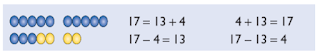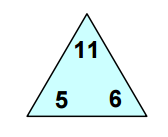### Really Understand What it Means to Add or Subtract

Children will be adding and subtracting numbers in many contexts throughout their lives. They will need to learn to add and subtract large numbers, decimals, fractions, negatives, algebraic equations and more. However, to be able to carry out these calculations with ease and understanding, students need to really get to grips with and fundamentally understand simple addition and subtraction at a young age. This post covers the early introduction to addition and subtraction with ideas for tasks that help children to really understand the process and the link between the two. To extend children further and develop a deeper understanding, including more on the commutative and associative property of addition (but not subtraction) and the issue with BIDMAS, I will shortly have a new post available.

(To see a full list of available posts click here.)

Addition is the process of finding the total when combining two or more quantities. It is considered to be one of the most simple numerical tasks and is taught to children from a very young age. To begin with, children are taught addition of single digit numbers using the decimal system and are then gradually extended on to more difficult concepts. There are two main ways of visually representing an addition problem; the first is to think of it as combining two sets (such as two piles of sweets for youngsters) and the second is to imagine extending an initial length by a second length (such as on a number line). Addition has a number of important properties:
• counting is the same as continually adding 1
• adding zero does not change the total
• addition is commutative - children need to understand that the order in which you add the numbers does not matter
3 + 5 = 8 and 5 + 3 =8
• addition is associative - when adding more than two numbers, it can help children if they realise that the order in which the addition is performed does not matter
4 + 3 + 7 = 4 + (3 + 7) = 14
Subtraction is the inverse of addition; subtracting a number 'undoes' the effect of adding the same number. It is the process of finding the difference between two quantities, or finding how many are left after taking one quantity away from another. Although addition and subtraction are strongly linked, the commutative and associative properties of addition do not apply to subtraction.

8 - 3 = 5 but 3 - 8 = -5
8 - 3 - 1= 4 but 8 - (3 - 1) = 6

I have seen many resources that try to overcome this problem by stating that you must always start with the larger number. Although usually correct for early subtraction problems, this is an incorrect statement and can lead to the deeply embedded misconception that you can not take a bigger number from a smaller. This statement is dangerous and should be avoided. Instead of telling children to always start with the larger number, talk about the numbers as parts and totals (for example with 3 + 5 = 8, 3 and 5 are the parts and 8 is the total) and then tell the children that they must always start with the total.

So much more than just adding or subtracting

A deep understanding of the basics will make moving on to more difficult concepts (such as adding/subtracting fractions or negatives) a much smoother process. For example:• A good understanding of the commutative property of addition is vitally important for future mathematics. Understanding that to answer 3 + 72, you can actually do 72 + 3 makes mental arithmetic much easier. It also enables for effective manipulation of algebraic equations, as students must understand that x + y + 2x is the same as x + 2x + y in order to simplify to 3x + y.
• Mathematical symbols (+, -, =) can seem like very abstract, irrelevant objects that are yet another thing to learn in a child's busy life. However, if children understand the meaning of (and the vocabulary associated with) adding and subtracting, they will be able to apply this to solving problems at a later point. I have seen many talented mathematicians who really struggle when interpreting worded problems and writing equivalent equations. If children can understand the mathematical symbols as shorthand ways of writing more meaningful phrases then they will more easily be able to set up their own equations.

Use language to emphasise meaning
Before presenting children with written equations that use mathematical symbols, ask them worded questions and use props that really require them to think about the process that they are using to arrive at the answer. Use language such as 'count on', 'how many altogether', 'how many more', 'how many fewer', 'what is the difference', etc.. Start with small, single digit numbers and mix together addition and subtraction problems so that children don't see them as completely separate topics to learn. Avoid using mathematical symbols until the children are confident with the process; this encourages them from the start to use addition and subtraction to solve 'real-life' problems, rather than the other way round.

Introduce the mathematical symbols
In order to understand and write addition and subtraction equations, children need to know the '+', '-' and '=' symbols. The '+' and '-' symbols can be introduced using the language from the last task but the '=' symbol needs special emphasis. I have seen the equals symbol used incorrectly by students of all ages and at all stages of learning. It is a fundamental principle that many students do not fully understand and it makes many other areas of mathematics a lot more difficult.

Encourage children to write mathematical equations from images and 'real-life' problems and use language that will help them understand and visualise the problem they are trying to solve. It is important to get children to write their own number sentences from pictures, rather than using pictures to help solve equations. For example, present children with a picture of two bunches of flowers and ask them for the total number of flowers. Encourage them to create their own equation to record the maths they have done in solving it. This encourages them to think of addition and subtraction equations as tools for solving and recording problems and will help them apply number sentences for future, more difficult problems. I found a lovely worksheet of TES that has nothing but pictures and encourages the children to write, and really understand, their own number sentences.

Sets of objects in two coloursPicture from the National Strategies
Give children a set of objects in two colours and ask them to make an equation or number sentence. You can then compare and discuss all the different answers. Some children will have made addition equations and some will have made subtraction ones: whichever each individual child gave, they should all be able to see how the picture could also represent the opposite. This emphasises the link between addition and subtraction, without you having to try and explain it. Children will, in their own way, be able to 'see' the relationship and may even be able to explain it to you.

This activity is also a great opportunity to highlight the commutative property of addition. Children will see that it doesn't matter that they had 13 + 4 but their friend had 4 + 13, they both ended up with 17. Again, the ability to physically 'see' this and come to the conclusion independently will help to really embed the concept and to be able to apply it in other situations. It also enhances awareness of why the corresponding subtraction facts start with the larger number (or total) without having to tell the students the incorrect fact that subtraction always starts with the larger number.

Number linesClick here to download board game

Although some of the other methods may be more visual and intuitive for young children, I believe that using a number line is an important concept for children to understand. Number lines can more easily (than physical objects) be used when extending addition and subtraction into decimals or when starting with a negative number. It would therefore be a great help if children were already used to using a number line for early stages of addition and subtraction.

There are many fun and engaging ways of using a number line for counting, such as making a board game or using a life-size number line drawn on the playground to walk up and down.

Number trio cards
Give children a set of number trio cards and ask them to explore the relationship between the numbers. When they have realised that the bottom two numbers add to make the top number you can start covering up different corners and asking the children to find the missing number. This online version at topmarks allows you to choose different number bonds or additions and then reveal the numbers in each corner.

This task really helps children to understand the link between addition and subtraction: in the picture, if you cover up the 6, children will realise that they need 6 more to make 11, as this is the difference between 11 and 5. By discussing the answers and using the appropriate language, children can see that they could have described what they were doing as an addition or as a subtraction.The task also emphasises the fact that it does not matter whether you add 5 to 6 or 6 to 5, as all you are doing is combining the two quantities at the bottom. However, it will become clear to the students that, when talking about the corresponding subtraction facts, they must always start with the top number. A task such as this uses numerals rather than objects and encourages children to start thinking about equations without yet requiring them to fully understand abstract symbols.

Use number/symbol cards
Give children a simple set of cards such as those shown in the picture. Ask them to make as many correct equations as they can. If they are initially struggling, give them a set of objects (in two colours for extra help). Encourage the children to record their results and explain their findings. If they can explain the link between addition and subtraction in their own words then they are much more likely to remember it and apply it to other situations

Frequent questions that require knowledge of the link between addition and subtraction
Start by asking simply questions such as

if 7 add 4 is 11, what is 11 take away 4?

Encourage children to use their knowledge of the link between addition and subtraction, rather than relying on working it out from scratch. Maybe use pictures to reinforce the knowledge for children who are struggling to grasp the concept. Once children are more confident with the idea, you can extend them with more difficult questions, such as

if 732 add 4627 is 5359, what is 5359 take away 732?

Clearly, you are not expecting children to calculate the answer by counting so you will be relying on their knowledge of the link between addition and subtraction to answer the question. This is a great way to truly test the children's understanding. I have known many secondary school students unable to give the correct answer without using written methods (such as column addition) or a calculator.

Many older children show signs of misconceptions and lack understanding of the process of addition and subtraction, and this makes their lives a lot more difficult in many areas of mathematics. I strongly believe that if they develop a deep and fundamental understanding of adding and subtracting early on, this can lead to a much more positive experience with maths throughout school.